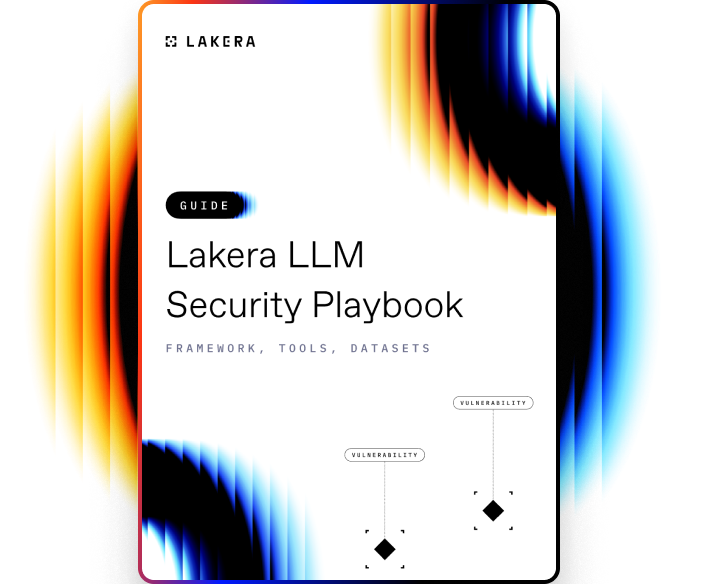VOCABULARY

# Batch Normalization

Batch normalization is a technique used in training deep neural networks to standardize the inputs to a layer for each mini-batch. This process helps to stabilize the learning process and dramatically reduces the number of training steps required to converge.

## How Batch Normalization works

Batch Normalization works by normalizing the output of a previous activation layer by subtracting the batch mean and dividing by the batch standard deviation. However, this could potentially lead to the network losing some of the representational power of the layer. To prevent this from happening, two parameters, scale (gamma) and shift (beta), are learned during the normalization process which allows the model to undo the normalization.

In short, the process of Batch Normalization can be broken down into the following steps:

1. It calculates the mean and variance of the layers' input.
2. It normalizes the layer inputs using the calculated mean and variance.
3. It scales and shifts the normalized inputs using the scale (gamma) and shift (beta) parameters.

The key benefit of Batch Normalization is that it makes neural networks more robust to the choice of initialization and learning rate. Moreover, it allows each layer of a network to learn on a more stable distribution of inputs, which can speed up the training process.Learn how to protect against the most common LLM vulnerabilities

Download this guide to delve into the most common LLM security risks and ways to mitigate them.

Related termsActivate
untouchable mode.